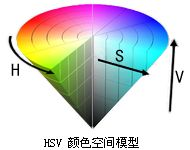# HSVtoRGB

This function is used to convert the HSV color to RGB color.

``````COLORREF HSVtoRGB(
float H,
float S,
float V
);
``````

## Parameters

### H

Hue component of the original HSV color model, 0<=H< 360.

### S

The Saturation component of the original HSV color model, 0<=S<= 1.

### V

The Value component of the original HSV color model, 0 <= V <= 1.

## Return Value

The corresponding RGB color.

## Remarks

HSV is also called HSB.

The color model of the HSV is shown:H is the first letter of the English Hue, which represents the hue, which is the monochrome that makes up the visible spectrum. Red is at 0 degrees, green is at 120 degrees, and blue is at 240 degrees, which transitions in this direction.

S is the first letter of the English Saturated, indicating saturation, which is equal to 0 when gray. At maximum saturation 1, each hue has the purest color light.

V is the first letter of the English Value, which represents the lightness, which is equal to 0 when black, and is the most distinctive state of color at the maximum lightness of 1.

## Examples

The HSV color model is similar to the HSL, and the "rainbow" in the sample program is an example of how the HSL model is operated and can be referenced.

（贡献者：Krissi  编辑